# Results of VisIt Regression Test - queries/xrayimage

 Test Case Errors Images %Diffs Maximum Baseline Current Diff Map xrayimage00 0.00 0.00xrayimage01 0 modifications totalling 0 lines xrayimage02 0.00 0.00xrayimage03 0.00 0.00xrayimage04 0 modifications totalling 0 lines xrayimage05 0.00 0.00xrayimage06 0 modifications totalling 0 lines xrayimage07 0.00 0.00xrayimage08 0 modifications totalling 0 lines xrayimage09 0.00 0.00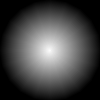xrayimage10 0 modifications totalling 0 lines xrayimage11 0.00 0.00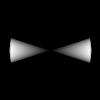xrayimage12 0 modifications totalling 0 lines xrayimage13 0.00 0.00xrayimage14 0 modifications totalling 0 lines xrayimage15 0.00 0.00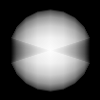xrayimage16 0 modifications totalling 0 lines xrayimage17 0.00 0.00xrayimage18 0 modifications totalling 0 lines xrayimage19 0.00 0.00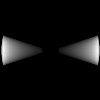xrayimage20 0 modifications totalling 0 lines xrayimage21 0.00 0.00xrayimage22 0 modifications totalling 0 lines xrayimage23 0.00 0.00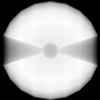xrayimage24 0 modifications totalling 0 lines xrayimage25 0.00 0.00xrayimage26 0 modifications totalling 0 lines xrayimage27 0.00 0.00xrayimage28 0.00 0.00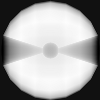xrayimage29 0 modifications totalling 0 lines xrayimage30 0.00 0.00xrayimage31 0 modifications totalling 0 lines xrayimage32 0 modifications totalling 0 lines Blueprint_HDF5_X_Ray_Output 0.00 0.00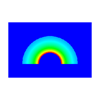Blueprint_HDF5_X_Ray_Output_Cycle ` 48.00000 .eq. 48.00000 (prec=5) : True` Blueprint_HDF5_X_Ray_Output_Normal ` ['0.00000', '0.00000', '1.00000'] .eq. ['0.00000', '0.00000', '1.00000'] (prec=5) : True` Blueprint_HDF5_X_Ray_Output_Focus ` ['0.00000', '2.50000', '10.00000'] .eq. ['0.00000', '2.50000', '10.00000'] (prec=5) : True` Blueprint_HDF5_X_Ray_Output_ViewUp ` ['-0.00000', '1.00000', '0.00000'] .eq. ['0.00000', '1.00000', '0.00000'] (prec=5) : True` Blueprint_HDF5_X_Ray_Output_ViewAngle ` 30.00000 .eq. 30.00000 (prec=5) : True` Blueprint_HDF5_X_Ray_Output_ParallelScale ` 5.00000 .eq. 5.00000 (prec=5) : True` Blueprint_HDF5_X_Ray_Output_NearPlane ` -100.00000 .eq. -100.00000 (prec=5) : True` Blueprint_HDF5_X_Ray_Output_FarPlane ` 100.00000 .eq. 100.00000 (prec=5) : True` Blueprint_HDF5_X_Ray_Output_ImagePan ` ['0.00000', '0.00000'] .eq. ['0.00000', '0.00000'] (prec=5) : True` Blueprint_HDF5_X_Ray_Output_ImageZoom ` 1.00000 .eq. 1.00000 (prec=5) : True` Blueprint_HDF5_X_Ray_Output_Perspective ` 0.00000 .eq. 0.00000 (prec=5) : True` Blueprint_HDF5_X_Ray_Output_SpatialExtents0 ` ['0.00000', '0.00000'] .eq. ['0.00000', '0.00000'] (prec=5) : True` Blueprint_HDF5_X_Ray_Output_SpatialExtents1 ` ['0.05000', '0.05000'] .eq. ['0.05000', '0.05000'] (prec=5) : True` Blueprint_HDF5_X_Ray_Output_SpatialExtents2 ` ['0.10000', '0.10000'] .eq. ['0.10000', '0.10000'] (prec=5) : True` Blueprint_HDF5_X_Ray_Output_SpatialExtents3 ` ['15.00000', '10.00000'] .eq. ['15.00000', '10.00000'] (prec=5) : True` xrayimage33 0 modifications totalling 0 lines Blueprint_JSON_X_Ray_Output 0.00 0.00xrayimage34 0 modifications totalling 0 lines Blueprint_YAML_X_Ray_Output 0.00 0.00xrayimage35 0 modifications totalling 0 lines xrayimage36 0 modifications totalling 0 lines Test_filenames_for_jpeg_outputs 0 modifications totalling 0 lines Test_filenames_for_png_outputs 0 modifications totalling 0 lines Test_filenames_for_tif_outputs 0 modifications totalling 0 lines Test_filenames_for_bof_outputs 0 modifications totalling 0 lines Test_filenames_for_bov_outputs 0 modifications totalling 0 lines Test_filenames_for_json_outputs 0 modifications totalling 0 lines Test_filenames_for_hdf5_outputs 0 modifications totalling 0 lines Test_filenames_for_yaml_outputs 0 modifications totalling 0 lines Test_filenames_for_family0_outputs 0 modifications totalling 0 lines Test_filenames_for_family1_outputs 0 modifications totalling 0 lines detect and warn numpy array as query param ` True .eq. True (prec=0) : True` numpy array converted to list works as query param ` True .eq. True (prec=0) : True`

Final Return Code: 111